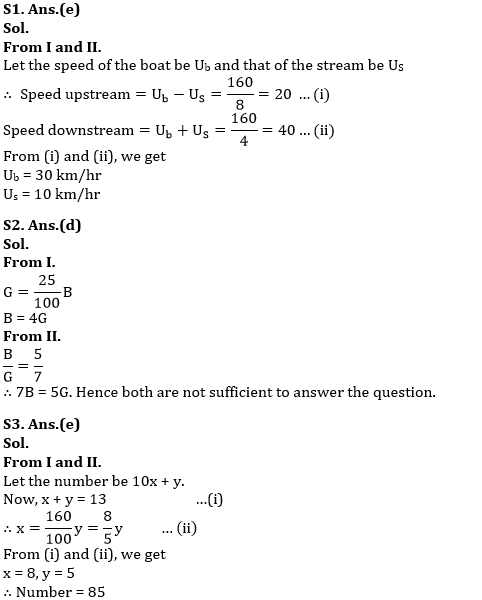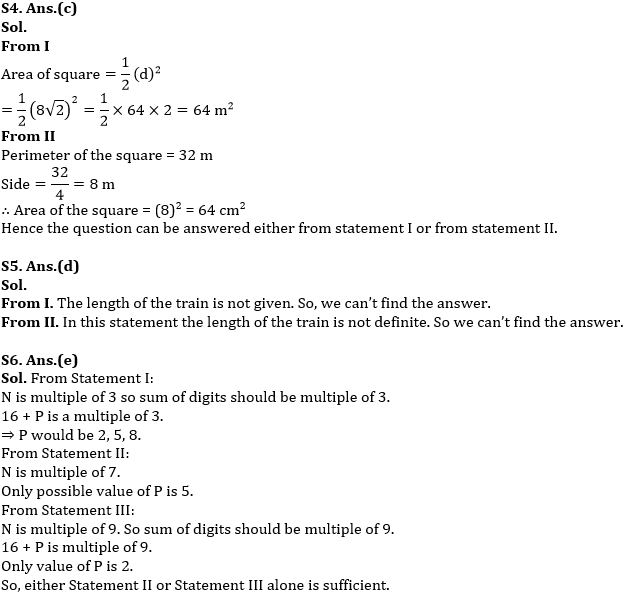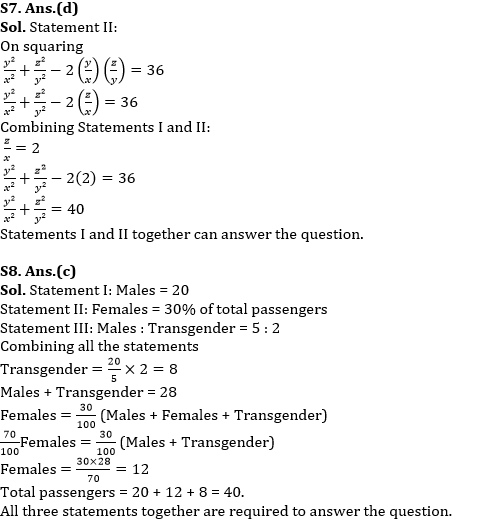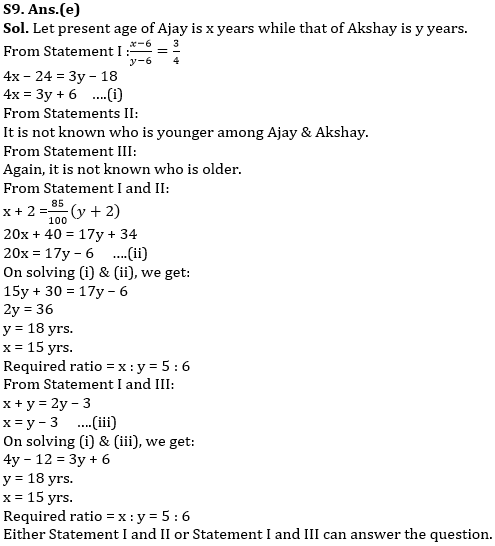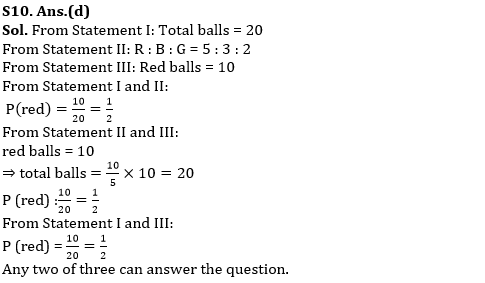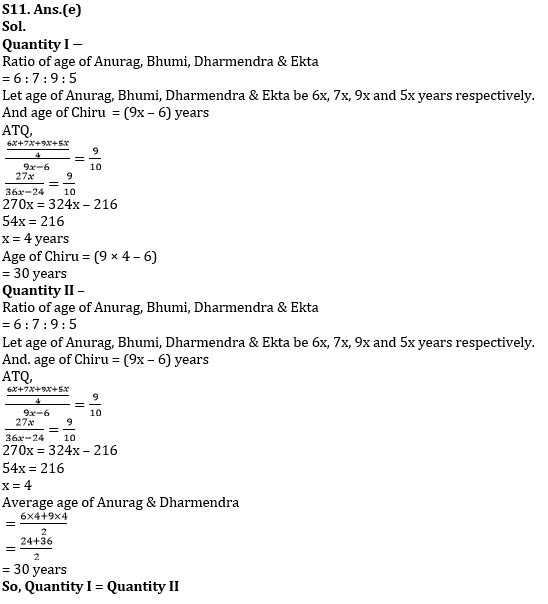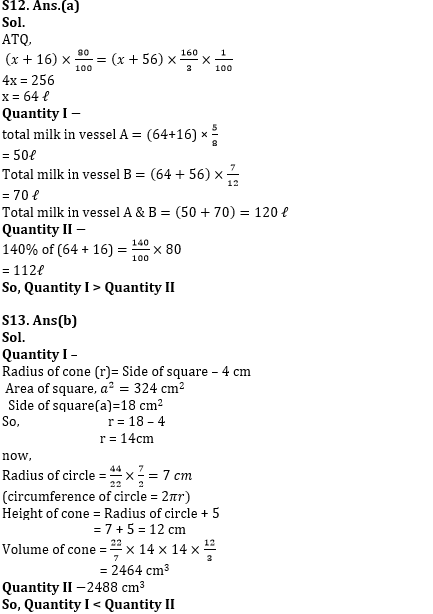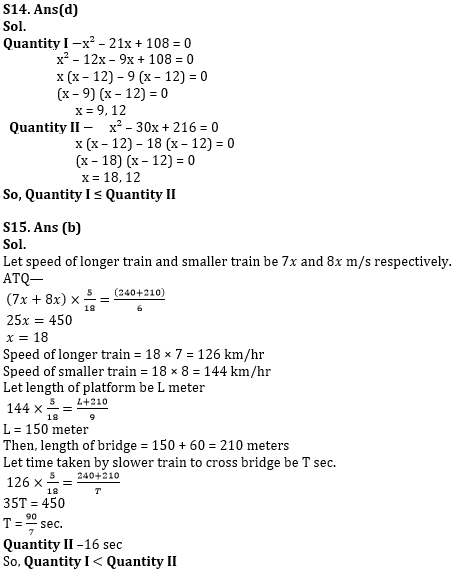Latest Banking jobs   »   Quantitative Aptitude Quiz For RBI Grade...

# Quantitative Aptitude Quiz For RBI Grade B/ ECGC PO/ SIDBI Grade A Prelims 2022- 27thApril

Directions (1-5): Each of the questions below consists of a question and two statements numbered I and II given below it. You have to decide whether the data provided in the statements are sufficient to answer the question. Read both the statements and give answer
(a) if the data in statement I alone are sufficient to answer the question, while the data in statement II alone are not sufficient to answer the question.
(b) if the data in statement II alone are sufficient to answer the question, while the data in statement I alone are not sufficient to answer the question.
(c) if the data either in statement I alone or in statement II alone are sufficient to answer the question.
(d) if the data in both statement I and II together are not sufficient to answer the question.
(e) if the data in both statement I and II together are necessary to answer the question.

Q1. What is the speed of a boat in still water ?
I. The boat covers a distance of 160 km in 8 hours while running upstream.
II. It covers the same distance in 4 hours while running downstream.

Q2. How many boys are there in the class ?
I. The number of girls is 25% of the number of boys.
II. The ratio of the number of boys to that of girls is 5 : 7.

Q3. What is the value of a two-digit number ?
I. The sum of the digits of the number is 13.
II. The digit at the tens place is 160% of the digit at the units place.

Q4. What is the area of a square ?
I. The diagonal of the square is 8√2 m.
II. The perimeter of the square is 32 m.

Q5. What is the speed of a train ?
I. The train crosses a pole in 16 seconds.
II. The train crosses a platform of equal length in 54 seconds.

Directions (6-10): Each of the following question is followed by three statements I, II and III. You have to study the question and the statements and decide which of the statements is/are necessary to answer the question.

Q6. What is the exact value of P where N = 2P347?
I. N is a multiple of 3.
II. N is a multiple of 7.
III. N is a multiple of 9.
(a) The data in statement I alone is sufficient.
(b) The data in statements I and II together is sufficient.
(c) The data in all the statements together is required.
(d) Any two of three statements are sufficient.
(e) either statement II or statement III alone can answer the question.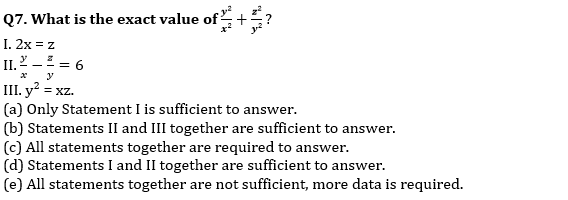Q8. How many people are there in the bus consisting male, female and transgender?
I. There are 20 males in the bus.
II. 30% of passengers are females.
III. The ratio of males to transgender is 5 : 2.
(a) Statements I and II together can answer the question.
(b) Statements II and III together can answer the question.
(c) All the given three statements together are required to answer the question.
(d) Any two statements can answer.
(e) All three statements together are not sufficient to answer the question, more data is required.

Q9. What is the ratio of the present age of Ajay and Akshay?
I. 6 years ago, ratio of age of Ajay to Akshay is 3 : 4.
II. After 2 years, the younger one’s age will be 85% of the older.
III. The sum of their ages is three less than twice the age of older.
(a) Only statements II and III together are sufficient.
(b) Any two of them is sufficient.
(c) Only statements I and II together are sufficient.
(d) All statements are not sufficient to answer.
(e) None of the above.

Q10. What is the probability of drawing a red ball from a bag containing red, blue and green balls?
I. There are total 20 balls in the bag.
II. The ratio of red, blue and green balls is 5 : 3 : 2.
III. There are 10 red balls in the bag.
(a) Only Statement II is sufficient.
(b) Only Statements I and II together are sufficient.
(c) Only Statements II and III together are sufficient.
(d) Any two of the three statements together.
(e) All statements are required to answer.

Directions (11-15): In the given questions, two quantities are given, one as ‘Quantity I’ and another as ‘Quantity II’. You have to determine relationship between two quantities and choose the appropriate option:

Q11. Ratio of age of Anurag and Bhumi is 6 : 7 and Dharmendra : Ekta is 9 : 5. Ratio of average age of Anurag, Bhumi, Dharmendra& Ekta to age of Chiru is 9 : 10
Quantity I- If ratio of age of Bhumi and Dharmendra is 7 : 9 and age of Chiru is 6 years less than age of Dharmendra, then age of Chiru.
Quantity II – If ratio of age of Bhumi and Dharmendra is 7 : 9 and age of Chiru is 6 years less than age of Dharmendra, Average age of Anurag& Dharmendra.
(a) Quantity I > Quantity II
(b) Quantity I < Quantity II
(c) Quantity I ≥ Quantity II
(d) Quantity I ≤ Quantity II
(e) Quantity I = Quantity II or no relation

Q12. Two vessels A & B contains (x + 16) l and (x + 56) l mixture of milk and water respectively. If 20% from mixture from vessel A &46⅔% mixture from Vessel B taken out remaining mixture in both vessels become equal.
Quantity I – If ratio of milk to water in vessel A & B is 5 : 3 & 7 : 5 respectively, then total quantity of milk in both vessel.
Quantity II – 140% of total quantity of mixture in vessel A.
(a) Quantity I > Quantity II
(b) Quantity I < Quantity II
(c) Quantity I ≥ Quantity II
(d) Quantity I ≤ Quantity II
(e) Quantity I = Quantity II or no relation

Q13. Radius of cone is 4 cm less than side of square of which area is 324 cm². Height of cone is 5 cm more than radius of circle, whose circumference is 44 cm.
Quantity I –Volume of cone.
Quantity II –2488 cm³
(a) Quantity I > Quantity II
(b) Quantity I < Quantity II
(c) Quantity I ≥ Quantity II
(d) Quantity I ≤ Quantity II
(e) Quantity I = Quantity II or no relation

Q14. Quantity I –x² – 21x + 108 = 0
Quantity II – x² – 30x + 216 = 0
(a) Quantity I > Quantity II
(b) Quantity I < Quantity II
(c) Quantity I ≥ Quantity II
(d) Quantity I ≤ Quantity II
(e) Quantity I = Quantity II or no relation

Q15. 240 meters & 210 meters long trains running in opposite direction cross each other in 6 sec. Ratio between speed of longer train and smaller train is 7 : 8 and faster train cross a platform in 9 sec.
Quantity I – Time taken (in sec) by slower train to cross a bridge, which is 60 meters longer than platform.
Quantity II –16 sec
(a) Quantity I > Quantity II
(b) Quantity I < Quantity II
(c) Quantity I ≥ Quantity II
(d) Quantity I ≤ Quantity II
(e) Quantity I = Quantity II or no relation

Solutions